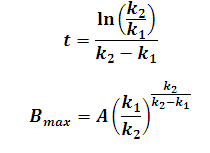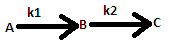# Maximum Yield of Intermediate Product In Sequential Reaction Calculator

This Calctown Calculator calculates the maximum yield of the intermediate product in a sequential reaction.

s-1
s-1
mol/L

#### Result

s
mol/LClick here to view image

where

Bmax = maximum yeild of B

t = time for Bmax

k1 = reaction rate of first reaction

k2 = reaction rate of 2nd reaction

A = initial concentration of A